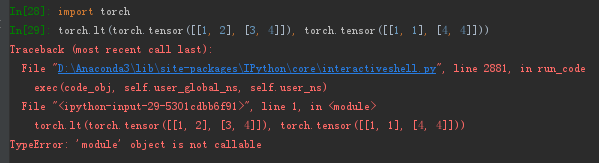# What is torch.lt for?

for the given script,
pattern = torch.lt(pattern,density):byte() – 25% 1s and 75% 0s (lua code)
what is the method torch.lt() for?

Hi,

You can find it in the doc here.

thanks for reply. but I can not run the given script in the doc ( torch.lt(torch.tensor([[1, 2], [3, 4]]), torch.tensor([[1, 1], [4, 4]])) ) ?besides, i do not understand the method very much according to the result.
why result return “tensor([[ 0, 0],
[ 1, 0]], dtype=torch.uint8)”

Which version of pytorch are you using? If you’re using an older version, use the doc for you version, the link I sent is for 0.4.

maybe 0.2. OK, thank you. can you explain the example, why the result is tensor([[ 0, 0],[ 1, 0]]).
is the method used for comparing two tensor?

Yes, it gives you a mask that contains 1 if the other tensor is bigger, 0 otherwise.

got it, thank you…